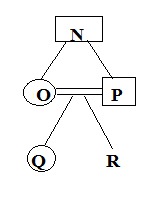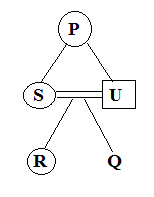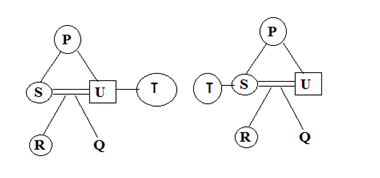# SSC CGL 2018 Practice Test Papers | Reasoning Ability (Day-26)

Dear Aspirants, Here we have given the Important SSC CGL Exam 2018 Practice Test Papers. Candidates those who are preparing for SSC CGL 2018 can practice these questions to get more confidence to Crack SSC CGL 2018 Examination.

[WpProQuiz 3054]

Click “Start Quiz” to attend these Questions and view Explanation

1) Mathematics: Logic:: Science: ?

a) Exercise

b) Laboratory

c) Fact

d) Scientist

2) ACF: CEH:: EGJ : ?

a) GIL

b) MIG

c) HOP

d) LMG

3) Find the odd one.

a) Wheat

b) Gram

c) Soya bean

d) Sugarcane

4) Find the odd one.

a) Mercury

b) Mars

c) Earth

d) Uranus

5) How many rectangles are there in the following figure?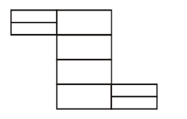a) 14

b) 18

c) 20

d) 22

6) In the following number series, how many ‘8’ are there which are preceded by ‘6’ and followed by ‘9’?

9 4 8 6 3 2 7 1 8 6 8 9 8 1 3 6 8 9 7 8 6 3 1 3 6 8 4 3 6 3 9

a) 2

b) 5

c) 3

d) 1

7)  If ‘+’ means divided by, ‘–’ means added to, ‘×’ means subtracted from, ‘÷’ means is multiplied by, then,

26 – 15 + 15 × 8 ÷ 2 =?

a) 23

b) 19

c) 11

d) 14

A+O means A is the mother of O

A-B means A is the father of B

A × D means A is the wife of D

A ÷ C means A is the sister of C

8) If N- O × P-Q ÷ R then which statement is true

a) N is the mother of O

b) N is the mother of R

c) O is the mother of Q

d) R is the son of O

9) If P+S×U-R÷Q then How P is the related to R?

a) Mother

b) Grandmother

c) Sister

d) Father

10) If T is the only Aunt of R, then how T is related to U as per the following equation P + S × U – R ÷ Q?

a) Sister

b) Sister in law

c) Either a or b

d) Mother

Mathematics is based on logic. Similarly, science is based on fact.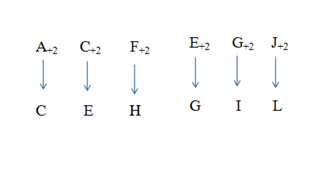Except option (D), all others are Rabi crops.

Except d all planets rotates in anticlockwise direction.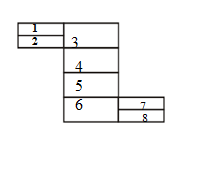Number of Rectangles= 1, 2, 3, 4, 5, 6, 7, 8, (1, 2), (3, 4), (5, 6), (4, 5), (7, 8), (1, 2, 3), (3, 4, 5),

(6, 7, 8), (4, 5, 6) and (3, 4, 5, 6)

26+15/15-8×2=11